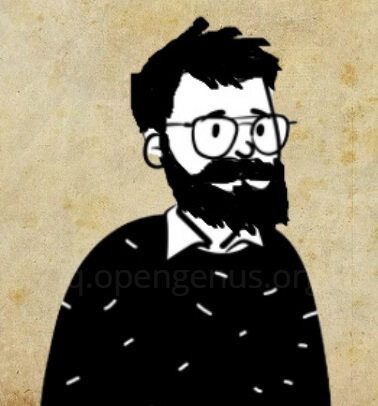×

Search anything:

# Convert std::vector<float> to float*

#### C++Get this book -> Problems on Array: For Interviews and Competitive Programming

In this article, we have explored 2 different approaches to convert `std::vector<float>` to float* in C++.

2. Approach 2: Get data
3. Approach 3: Create a copy

The key idea in this approach is to create a new float pointer (float*) and point it to the address of the first element in vector. This way both float vector and float array point to the same data.

This is the most efficient approach. This is the code snippet for conversion:

``````std::vector<float> vector1(100);
float *array1 = &vector1;
``````

Following is the complete C++ working code:

``````#include <iostream>
#include <vector>

int main() {
std::vector<float> vector1(100);
float *array1 = &vector1;
return 0;
}
``````

## Approach 2: Get data

In this approach, we get the data of vector using data() method and assign it to vector array / pointer. Following is the code snippet for the approach:

``````std::vector<float> vector1(100);
float *array1 = vector1.data();
``````

The data method was introduced in C++11 and returns the direct pointer to the vector.

Following is the complete C++ working code:

``````#include <iostream>
#include <vector>

int main() {
std::vector<float> vector1(100);
float *array1 = vector1.data();
return 0;
}
``````

## Approach 3: Create a copy

The previous approaches share the elements between the vector and array. If you wish to make the array point to a copy of the elements of the vector, the approach is to create a float array (same as float pointer) and use copy method.

``````float array1;
std::copy(vector1.begin(), vector1.end(), array1);
``````

Following is the complete C++ working code:

``````#include <iostream>
#include <vector>

int main() {
std::vector<float> vector1(100);
float array1;
std::copy(vector1.begin(), vector1.end(), array1);
return 0;
}
``````

With this article at OpenGenus, you must have the complete idea of how to convert `std::vector<float>` to float*.#### Geoffrey Ziskovin

Geoffrey Ziskovin is an American Software Developer and Author with an experience of over 30 years. He started his career with Haskell and has interviewed over 700 candidates for Fortune 500 companies

Convert std::vector<float> to float*